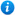* Watermarks do not appear on purchased resources

Preview resource: Click slides to enlarge

The Four Operations - Year 6

The Four Operations - Year 6

Year 6 maths programme of study - Number (addition, subtraction, multiplication and division):

• multiply multi-digit numbers up to 4 digits by a two-digit whole number using the formal written method of long multiplication
• divide numbers up to 4 digits by a two-digit number using the formal written method of short division where appropriate, interpreting remainders according to the context
• solve addition and subtraction multi-step problems in contexts, deciding which operations and methods to use and why
• use their knowledge of the order of operations to carry out calculations involving the four operations
• solve problems involving addition, subtraction, multiplication and division

This Year 6 maths teaching resource includes a PowerPoint and differentiated worksheets for independent learning, to guide your class through using the four operations to solve calculations and multi-step problems as per the curriculum objectives listed above. It will help the children understand how to solve addition and subtraction problems in context, along with developing their understanding of long multiplication and short division. By exploring the four operations, the resource helps children learn which operation to use and why. The worksheets contain varied fluency, reasoning and problem solving to reinforce and consolidate the children's learning. The resource contains five lessons, which support the White Rose Small Steps guidance, and are designed to support a mastery approach to teaching maths.

Content includes:

1. Lessons 1 and 2: Addition and Subtraction with 2 accompanying worksheets with answers
2. Lesson 3: Long Multiplication with 1 accompanying worksheet with answers
3. Lesson 4: Short Division with 1 accompanying worksheet with answers
4. Lesson 5: Consolidation of skills learned so far with challenge cards

'The Four Operations - Year 6' is completely editable giving teachers the freedom to adapt the resource to suit their individual teaching needs.

Click on the images from the PowerPoint presentation to view the resource in more detail.

Our Price : £5.99 / 6 Credits